# Python: Arithmetic, Relational Operators, and Variables

## Arithmetic Operators

Python Arithmetic Operators are pretty standard.

## Arithmetic Operators

• – : Subtraction
• * : Multiplication
• / : Division
• //: Integer Division (Return divisor: 5/2 = 2)
• % : Modulus (returns the remainder of a division problem: 5%2=1)
• ** : Exponent (4**2 = 16)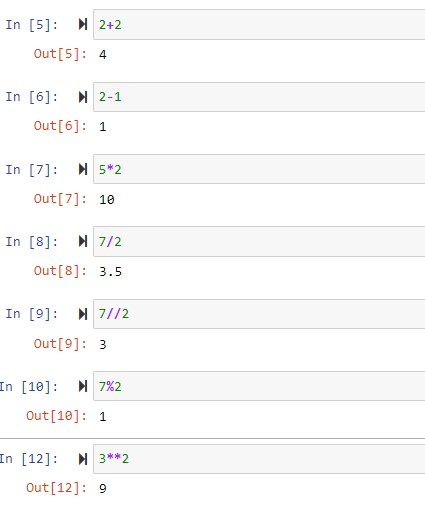Try your code in our online Python console:## Relational Operators

Relational operators compare values and return a Boolean result (Boolean means 2 states, in this case True or False). Note when using relational operators, == is equal to. A single = is used to set a variable value, not to compare two values

> Greater than

< Less Than

== Equal to

!= not equal

>= or <= greater than or equal, less than or equal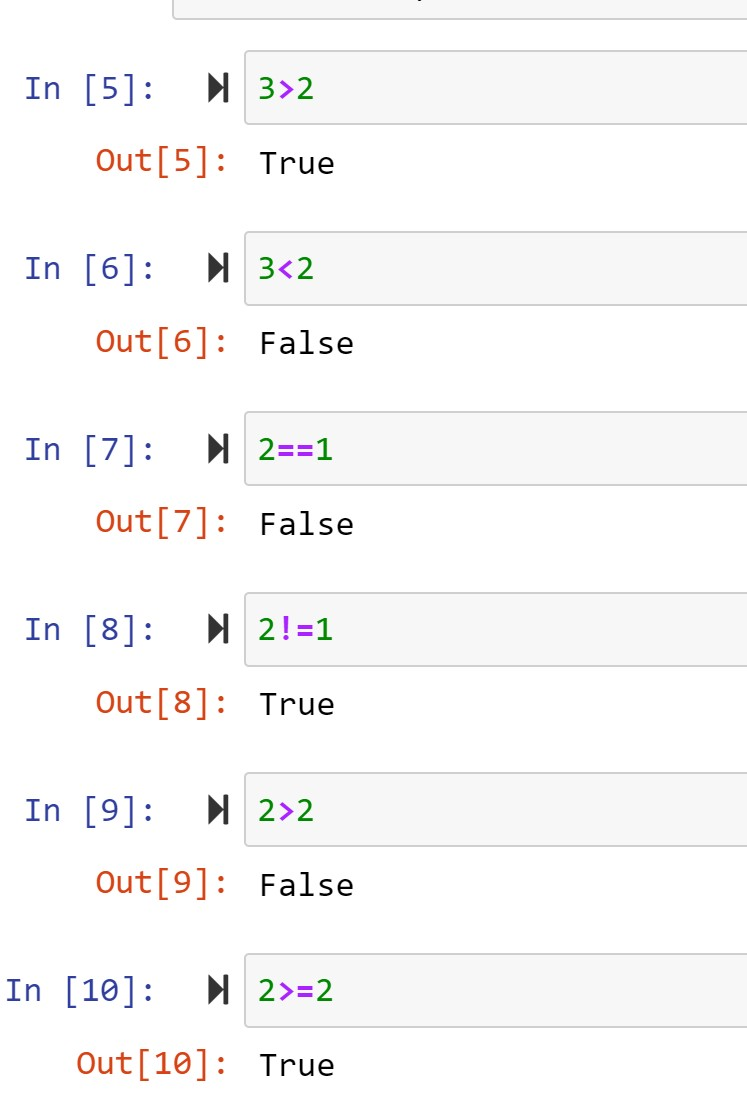Try your code in our online Python console:## Variables

Variables are a way of holding values in a program. You take a unique character string and you can assign values to it, and use the string throughout your program. Variables in Python are pretty straight forward. Unlike other programming languages, you do not need to define the variables first. Python dynamically assigns the data type.

Three main rules:

• Variables are case sensitive
• Avoid using command keywords (print, def, for)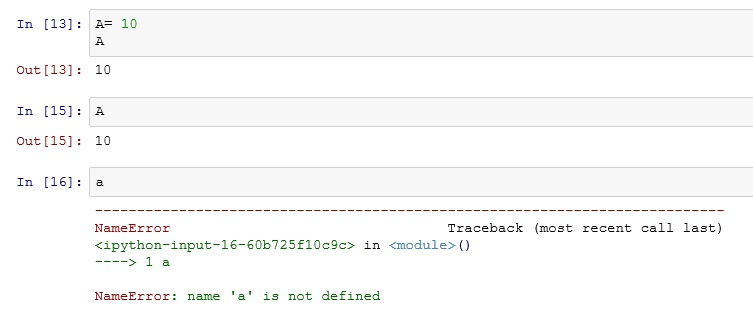notice lowercase ‘a‘ returns an error

Remember, Jupyter notebooks only return the last command. If you want both variables, use the print command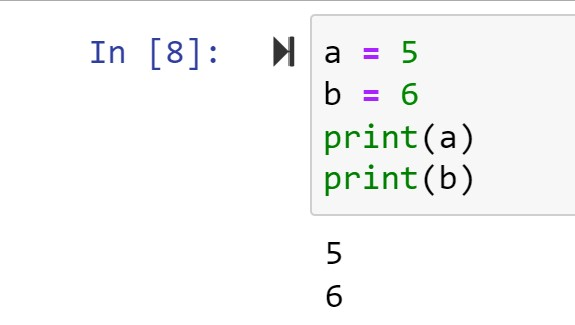You can perform arithmetic functions on variablesAnd of course, variables can hold strings as well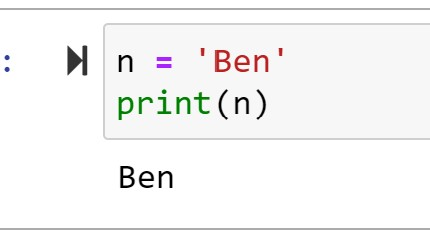Try your code in our online Python console:Last Lesson: Install Python and Hello World

Next Lesson: Print Variables and User Input

Back to Python Course: Course

## 2 thoughts on “Python: Arithmetic, Relational Operators, and Variables”

1.archer920gmailcom

This is a great demonstration of python variables and arithemtic operators! Thank you for the post!

2.86Deborah

I have noticed you don’t monetize your blog, don’t waste your traffic, you can earn extra bucks every month because you’ve got hi quality content.
If you want to know how to make extra money, search for: best adsense alternative Wrastain’s
tools